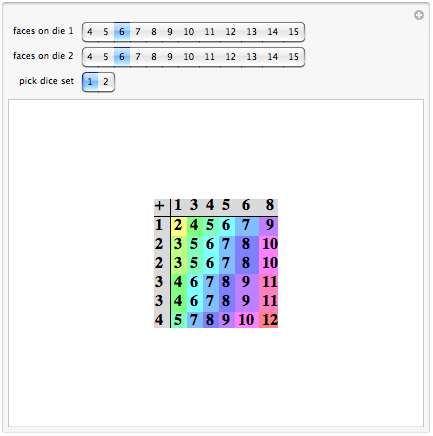Browse by Topic
Related Topics

# Sicherman Dice

Is it possible to have a pair of nonstandard dice with the same odds as regular dice?

Sure. You just need to know how to calculate the odds, and how to determine what different numbers could be on the faces to give the same odds. Let’s start with some tables.

The addition table is one of the first tables learned in school. Here is
one way to present an addition table in Mathematica.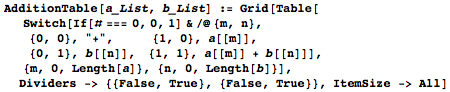The addition table can be used to examine the values obtained by rolling two standard dice. For example, rolling a 7 occurs six times in the table. A 12 occurs once. The number of times each value occurs is known as the distribution.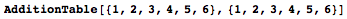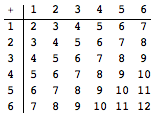If the two dice have different values on their faces, an addition table can be used to see the new distribution. Note, though, that the distribution of values in this new set and the two standard dice are identical—the numbers are the same, they’ve just been moved around.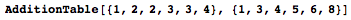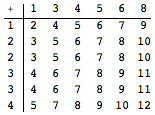That’s kind of neat, maybe. But how was it found? For that, a multiplication table will be useful.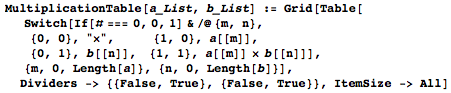Instead of integers, we’re using powers of x to multiply together. As might be expected, when two powers of an identical variable are multiplied, the powers add. Thus, a multiplication table can look something like an addition table.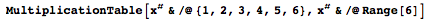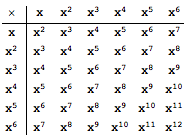Another way to find the distribution is to transform each set of values into a polynomial, and then to multiply the polynomials together. Both sets of dice above give the same polynomial.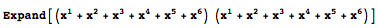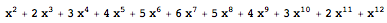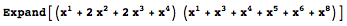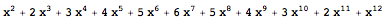One method for finding an alternative set of dice for a given distribution is to factor it.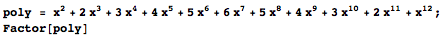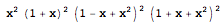Ideally, code is needed that gives all the polynomial divisors with a coefficient sum of 6, as each of the dice has 6 faces. The following Mathematica code accomplishes that.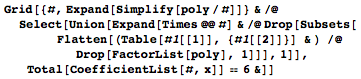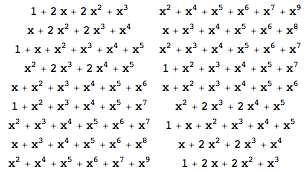These alternative distributions were brought to the attention of Martin Gardner by George Sicherman in 1978. More explorations of dice distributions can be found at the Sicherman Dice Demonstration.